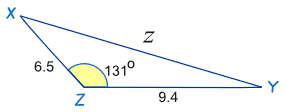D

#### Find the distance "z"

2 viewed last edited 3 years agoAnonymous
0Krishna
0

Step 1: Recall The Law of Cosinesab and c are sides.

C is the angle opposite side c

The Law of Cosines (also called the Cosine Rule) says:

c^2 = a^2 + b^2 - 2ab cos (C)

Step 2: Look at the figure and note down the given values.

Step 3: Substitute the known values in the Cosine rule.

EXAMPLE: c^2 = a^2 + b^2 - 2ab cos (C)

z^2 = (9.4)^2 + (6.5)^2 - 2(9.4)(6.5) cos (131)

Step 4: Do some calculations

z^2 = 88.36 + 42.25 - 122.2 * (-0.656)

z^2 = 130.61 + 80.17

Step 5: Apply the square root on both sides. To know the unknown length.

z^2 =210.78

z = \sqrt{210.78}

z = 14.5